# Introduction

The LEGENDplex system from BioLegend, the CBA system from BD Biosciences, and the MACSPlex from Miltenyi Biotec are all bead based multiplex system for simultaneous analysis of several analytes. The systems differ slightly but they all use beads coated with capture antibodies against analytes of interest, such that one bead is coated with one type of antibodies. After incubation with the sample (usually serum or a supernatant), the captured analytes are marked with a secondary antibody, much like a standard single analyte ELISA, and finally detected with a fluorochrome conjugated antibody against the secondary antibody. The type of analyte captures is identified by the properties of the beads. The individual assays are described below.

LEGENDplex

Beads fall into two large groups based on forward and side scatter properties. Within each group, the individual analytes detected are discriminated by the intensity of Allophycocyanin (APC) of the beads. The concentration of the analyte is related to the intensity of Phycoerythrin (PE).

CBA

All beads have similar forward and side scatter properties. The individual analytes are are discriminated by the intensity of APC and APC-Cy7 of the bead. The concentration of the analyte is related to the intensity of PE.

MACSPlex

All beads have similar forward and side scatter properties. The individual analytes are are discriminated by the intensity of PE and Fluorescein isothiocyanate (FITC) of the bead. The concentration of the analyte is related to the intensity of APC.

All multiplex systems come with their own analysis software. The analysis solutions come with a price tag or allows analysis of only a fixed number of beads. In addition, the usability and flexibility of the analysis solutions are restricted, and often impractical for experiments with a large number of samples.

Here the general usage of the beadplexr package is introduced. It will be demonstrated how to load the FACS-files, identify bead populations, draw standard curves and calculate concentrations of analytes.

## Naming LEGENDplex data-files

Each sample in the LEGENDplex experiment must have a unique and meaningful name. I suggest that the file name includes indication of the kit and the standard or experimental sample. For the indication of kit I use ‘K’ followed by a number, for the indication of the standard sample I use ‘C’ followed by a number – just as in the kit manual – and for the experimental sample, I use ‘S’ followed by a number. The different parts of the file name should be separated by a character not used in the IDs; this will make for easy parsing of the file names. It is not strictly needed, but adding a leading ‘0’ to all values below 10 will result in expected order when the files are sorted. If might be useful to include a ‘P’ followed by a number to indicate the plate number.

With this outlined nomenclature I can have the following FACS-files:

• K01-P01-C0-H1.fcs
• K01-P01-C01-G1.fcs
• K01-P01-C07-A1.fcs
• K01-P01-S01-A3.fcs
• K01-P01-S02-B4.fcs

Single letter abbreviations gives short file names and thereby reducing the chance of copy problems because of file paths being too long. The drawback is that a table must be kept which associates the kit ID with the actual kit name, and the sample ID with the actual sample name. However, in my experience, this is necessary when preparing for the experiment and does not bring much added work.

See the vignette Preparing flow-data for use with with beadplexr.

Each LEGENDplex panel measures different analytes, and the start concentration of the standards occasionally have different initial concentrations. The beadplexr package comes with all the required information for the LEGENDplex panels from BioLegend. These panels are loaded easily by passing the name or a name pattern to the load_panel() function:

library(beadplexr)
panel_info <- load_panel(.panel_name = "Human Growth Factor Panel (13-plex)")
panel_info$panel_name ##  "Human Growth Factor Panel (13-plex)" # Is equavalent to panel_info <- load_panel(.panel_pattern = ".*rowth.*panel") panel_info$panel_name
##  "Human Growth Factor Panel (13-plex)"

It is also possible to specify a file outside the package. The information file is in YAML format, and contains name and start concentration for each bead ID - grouped by major the bead groups - in addition to the name of the panel, the fold dilution of the standards, and the units of the analytes. The content of the YAML file for “Human Growth Factor Panel (13-plex)” looks like this:

## panel_name: Human Growth Factor Panel (13-plex)
## species: Human
## panel_manual: https://www.biolegend.com/media_assets/pro_detail/datasheets/Human_Growth_Factor_Panel_04272015.pdf
## analyte_unit: pg/ml
## std_dilution: 4
##
## # The beads fall on some major groups - for legendplex panels
## # there are two named A and B. The group_order element sets the
## # order of the major groups in the forward and side scatter channels.
## # In practice, then the groups are identified, the mean of the
## # forward satterchannel and the mean of the side scatter chennel
## # is calculated for each identified group and sorted by FSC and SSC
## group_order:
## - A
## - B
##
## # A list of Bead IDs,
## # where each bead ID consist of a list with Target name
## # and standard start concentration as value
## analytes:
##   A:
##     A4:
##       name: Angiopoietin-2
##       concentration: 50000
##     A5:
##       name: EGF
##       concentration: 10000
##     A6:
##       name: EPO
##       concentration: 50000
##     A7:
##       name: FGF-basic
##       concentration: 50000
##     A8:
##       name: G-CSF
##       concentration: 50000
##     A10:
##       name: GM-CSF
##       concentration: 50000
##   B:
##     B2:
##       name: HGF
##       concentration: 50000
##     B3:
##       name: M-CSF
##       concentration: 10000
##     B4:
##       name: PDGF-AA
##       concentration: 50000
##     B5:
##       name: PDGF-BB
##       concentration: 50000
##     B6:
##       name: SCF
##       concentration: 10000
##     B7:
##       name: TGF-a
##       concentration: 50000
##     B9:
##       name: VEGF
##       concentration: 50000

The panel information files bundled in the package are found in the directory /resources/ of the package directory. To find the package directory, simply issue:

system.file(package = "beadplexr")

## Get analyte MFI

The first step of the experiment analysis is to get the mean fluorescence intensity (MFI) of each analyte. This requires the identification of each analyte per size and APC intensity and the MFI of in the PE channel.

The beadplexr package comes with a small experiment already read into a list of data.frame. The data are from a “Human Growth Factor Panel (13-plex)” LEGENDplex experiment, with 8 controls and 1 human serum sample, all in duplicates. The beads were measured on a CytoFLEX cytometer, and the fcs-files were processed using read_fcs(), with default settings.

### Finding the analysis settings

It might get a bit of trying to get the settings correct for an experiment, but once established it should remain stable, provided that there are no change of cytometer, and that there is no particular drift in the used cytometer.

library(beadplexr)
library(dplyr)
library(purrr)

data(lplex)

#### Bead groups A and B

First task is to identify the two bead populations in the forward-side scatter. The package comes with a convenience function called identify_analyte(), which is a wrapper around

• Finding analyte clusters
• Trimming the clusters by removing the cluster members most distant from the cluster center
• Sorting the analyte clusters based on their centers
• Applying the population names A and B
plex_sub_sample <- lplex[]
plex_sub_sample <- identify_analyte(plex_sub_sample, .parameter = c("FSC-A", "SSC-A"),
.analyte_id = c("A", "B"),
facs_plot(plex_sub_sample, .x = "FSC-A", .y = "SSC-A", .beads = "Bead group")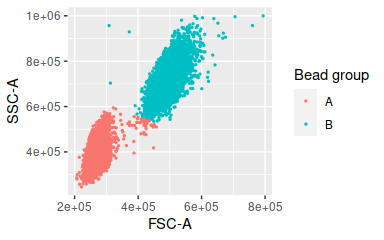The population names are assigned according to the size and side scatter so that the smallest population is given the first element of .analyte_id - the order of IDs is very important.

The above call use the default function clara (clustering large applications) from the package cluster. The package also provides convenience functions for kmeans and dbscan from the fpc package. Of the three I have found kmeans to do the worst, but it is included by public demand. dbscan is great for the forward-side scatter population identification, but requires some (and sometimes a lot) trial and error to get the parameters right. In addition it is a little slow compared to claraand kmeans. I have found that clara generally does a great job, however, for forward side scatter separation mclust might do better, although the model-based clustering at times is slower than clara.

The two populations above are quite well defined, but they include too much noise for my taste. Also, some events of the B bead group are assigned to the A group. It is not a lot of events that probably does not affect the outcome much, but we can do better.

We can either apply the dbscan or just remove the events furthest from the centers of the groups by setting the .trim parameter. In this case we remove 3% of the of the events based on their distance to the group center. The population center is found by a Gaussian kernel estimate.

plex_sub_sample$Bead group <- NULL plex_sub_sample <- identify_analyte(plex_sub_sample, .parameter = c("FSC-A", "SSC-A"), .analyte_id = c("A", "B"), .column_name = "Bead group", .trim = 0.03) facs_plot(plex_sub_sample, .x = "FSC-A", .y = "SSC-A", .beads = "Bead group")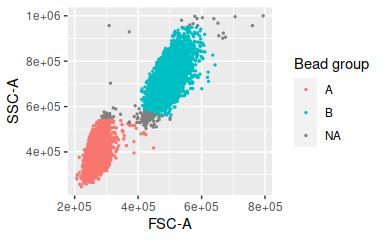#### Bead IDs The next task is to identify the individual beads within each of the bead groups. The analyte IDs for the “Human Growth Factor Panel (13-plex)” bead group A are A4, A5, A6, A7, A8, A10 and for group B B2, B3, B4, B5, B6, B7, B9. In this case, the beads are arranged from low to high, that is the lowest analyte ID has lowest intensity in the APC channel. library(ggplot2) panel_info <- load_panel(.panel_name = "Human Growth Factor Panel (13-plex)") bead_a <- plex_sub_sample |> filter(Bead group == "A") bead_b <- plex_sub_sample |> filter(Bead group == "B") bead_a <- identify_analyte(bead_a, .parameter = c("FL6-H"), .analyte_id = names(panel_info$analytes$A), .column_name = "Analyte ID") bead_b <- identify_analyte(bead_b, .parameter = c("FL6-H"), .analyte_id = names(panel_info$analytes$B), .column_name = "Analyte ID") # Factors are added for nicer plotting bead_a[["Analyte ID"]] <- factor(bead_a[["Analyte ID"]], levels = names(panel_info$analytes$A)) bead_b[["Analyte ID"]] <- factor(bead_b[["Analyte ID"]], levels = names(panel_info$analytes$B)) # Since the plot functions return a ggplot, we can easily add to these facs_density1d(bead_a, .x = "FL6-H", .beads = "Analyte ID") + ggtitle("Group A") facs_scatter(bead_a, .x = "FL2-H", .y = "FL6-H", .beads = "Analyte ID") + ggtitle("Group A") facs_density1d(bead_b, .x = "FL6-H", .beads = "Analyte ID") + ggtitle("Group B") facs_scatter(bead_b, .x = "FL2-H", .y = "FL6-H", .beads = "Analyte ID") + ggtitle("Group B")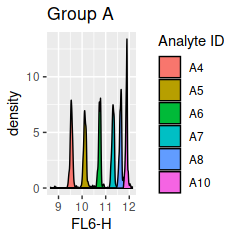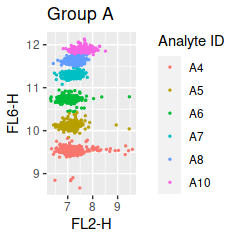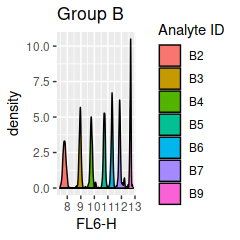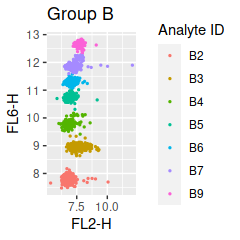The analytes are identified well, but there is a little too much noise, so we trim each analyte by using the function trim_population(). We can do this in the PE channel alone, but the B7 also needs trimming in the APC channel, so we trim in both PE and APC. bead_a |> ( function(x) split(x, list(x$Analyte ID))
)() |>
map_df(trim_population, .parameter = c("FL6-H", "FL2-H"),
.column_name = "Analyte ID",
.trim = 0.1) |>
# The trim_population removes all factors
mutate(Analyte ID = factor(Analyte ID, levels = names(panel_info$analytes$A))) |>
facs_scatter(.x = "FL2-H", .y = "FL6-H", .beads = "Analyte ID") + ggtitle("Group A")

function(x)
split(x, list(x$Analyte ID)) )() |> map_df(trim_population, .parameter = c("FL6-H", "FL2-H"), .column_name = "Analyte ID", .trim = 0.1) |> # The trim_population removes all factors mutate(Analyte ID = factor(Analyte ID, levels = names(panel_info$analytes$B))) |> facs_scatter(.x = "FL2-H", .y = "FL6-H", .beads = "Analyte ID") + ggtitle("Group B") ### Putting it all together It is tiresome to perform the above on each element of the FACS data list, and though you could wrap the code in a function to apply to each element of the FACS data list, it is much easier to use the built-in identify_legendplex_analyte(). panel_info <- load_panel(.panel_name = "Human Growth Factor Panel (13-plex)") args_ident_analyte <- list(fs = list(.parameter = c("FSC-A", "SSC-A"), .column_name = "Bead group", .method = "mclust", .trim = 0.03), analytes = list(.parameter = "FL6-H", .column_name = "Analyte ID")) analytes_identified <- identify_legendplex_analyte(df = lplex[], .analytes = panel_info$analytes,
.method_args = args_ident_analyte) |>
group_by(Analyte ID) |>
do(trim_population(., .parameter = c("FL6-H", "FL2-H"),
.column_name = "Analyte ID",
.trim = 0.1))

analytes_identified |> facs_plot(.x = "FSC-A", .y = "SSC-A", .beads = "Bead group")

analytes_identified |>
filter(Bead group == "A") |>
facs_plot(.x = "FL2-H", .y = "FL6-H", .beads = "Analyte ID")

analytes_identified |>
filter(Bead group == "B") |>
facs_plot(.x = "FL2-H", .y = "FL6-H", .beads = "Analyte ID")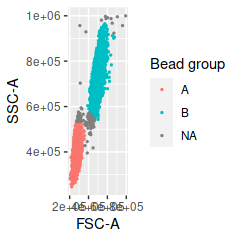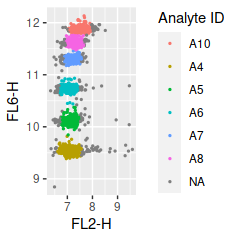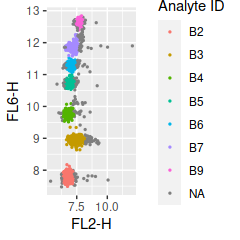This we can apply to the whole list, but before we start we create a little helper to identify the analytes and trim on FL6-H and FL2-H.

find_and_trim <- function(df){
identify_legendplex_analyte(df, .analytes = panel_info$analytes, .method_args = args_ident_analyte) |> group_by(Analyte ID) |> do(trim_population(., .parameter = c("FL6-H", "FL2-H"), .column_name = "Analyte ID", .trim = 0.1)) } analytes_identified <- lplex |> lapply(find_and_trim)  The FACS data is best visualized when the three dot-plots are side by side. library(gridExtra) plot_side_by_side <- function(df, .cur_sample){ plot_all_beads <- df |> facs_plot(.x = "FSC-A", .y = "SSC-A", .beads = "Bead group") + ggtitle("All events") plot_a_beads <- df |> filter(Bead group == "A") |> facs_plot(.x = "FL2-H", .y = "FL6-H", .beads = "Analyte ID") + ggtitle("Bead group A") plot_b_beads <- df |> filter(Bead group == "B") |> facs_plot(.x = "FL2-H", .y = "FL6-H", .beads = "Analyte ID") + ggtitle("Bead group B") arrangeGrob(plot_all_beads, plot_a_beads, plot_b_beads, nrow = 1, ncol = 3, top = .cur_sample) } analytes_identified |> ( function(x) map2(x, names(x), plot_side_by_side) )() |> walk(grid.arrange)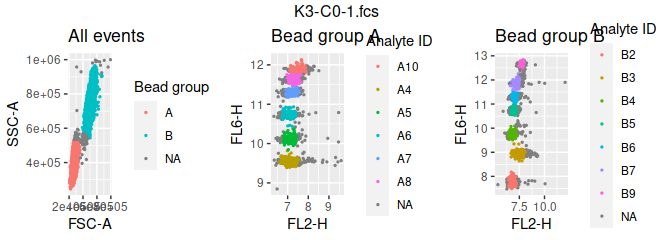Usually an experiment creates many files and it is probably better to save all the plots on a pdf file. all_plots <- analytes_identified |> ( function(x) map2(x, names(x), plot_side_by_side) )() |> marrangeGrob(ncol = 1, nrow = 4, top = NA) ggsave(filename = "dot_plot.pdf", plot = all_plots, width = 8.27, height = 11.69) Now with the analytes identified and the bead populations documented we can finally calculate the MFI of each analyte. beadplexr provides the possibility to calculate geometric, harmonic, and arithmetic mean. We combine the list of FACS data to a data.frame with Sample, Analyte ID, and the MFI because the creation of a standard curve in the next steps needs the MFI of several standard samples. analyte_mfi <- analytes_identified |> map_df(calc_analyte_mfi, .parameter = "FL2-H", .column_name = "Analyte ID", .mean_fun = "geometric", .id = "Sample") |> mutate(FL2-H = log10(FL2-H)) |> filter(!is.na(Analyte ID)) ## Calculate analyte concentration The calculation of the analytes requires two steps: 1. Create a standard curve by fitting a function to the MFI of the standard analytes and their known concentration. 2. Estimate the function of each sample analyte from the fitted function. Split the data.fame with the MFI information into standard and samples. library(stringr) # All standard samples have the pattern C[number] standard_data <- analyte_mfi |> filter(str_detect(Sample, "C[0-9]")) # All non standards are samples... we could also filter on S[number] sample_data <- analyte_mfi |> filter(!str_detect(Sample, "C[0-9]"))  The first thing needed to calculate the standard curve for each analyte is the concentration of the analyte. This we can calculate using the function calc_std_conc(), when we know the order of the samples, the start concentration, and the dilution factor. The order of the samples usually range from 0 to 7. If you follow the nomenclature on the LEGENDplex assay protocol 7 indicate the highest concentration of the standard analyte, 1 indicate the lowest concentration and 0 indicate no analyte. The start concentration for each analyte is stored in the Panel Information (depending on the panel it might differ from analyte to analyte). The dilution factor is also given in the Panel Information and is usually 4 (the concentration of each standard analyte is 4 times lower than the previous concentration). If - for some reason - you do not use the same dilution factor throughout all analytes and standards you need to indicate the appropriate dilution factors by hand. # Helper function to extract the sample number as_numeric_standard_id <- function(.s){ .s |> str_extract("C[0-9]") |> str_sub(start = -1L) |> as.numeric() } standard_data <- standard_data |> mutate(Sample number = as_numeric_standard_id(Sample)) |> left_join(as_data_frame_analyte(panel_info$analytes), by = "Analyte ID") |>
group_by(Analyte ID) |>
mutate(
Concentration = calc_std_conc(
Sample number,
concentration,
.dilution_factor = panel_info$std_dilution ) ) |> mutate(Concentration = log10(Concentration)) |> select(-concentration, -Bead group) The next step is to fit a standard curve for each analyte. With the standard curve we can calculate the concentration of the experimental samples (the purpose of the initial work), we can check the quality of the measurements - and the standard curve - by back calculate the standard concentration and compare this to the expected concentration, and we can plot the experimental samples on the standard curve (beadplexr provides easy functions for all of this). However, in each case we need to ensure that the correct standard curve is used with the correct data, which means we have to juggle at least three structures: A data.frame with the standard data, a data.frame with the experimental sample data and the models for each analyte (probably a list). It quickly become tedious to ensure that everything is in the correct order - and to be sure it is error prone. Luckily, the tidyr package provides the nest() and its inverse unnest() functions. It is arguably slightly odd to store complex objects in a data.frame, but it makes sense when you have several objects that logically belong together. It can take some time to get used to the changed view of the data.frame-structure, and it might help to remind yourself that a data.frame, really is a list where each column is an element of the list, and that there is no limitation to the type of objects stored in a list. library(tidyr) # It seems that tidyr::nest has problems with non-standard names, so the names # must all be concerted to syntactically valid column names. standard_data <- standard_data |> ungroup() |> ( function(x) setNames(x, make.names(names(x))) )() |> nest(Standard data = c(-Analyte.ID, -name)) sample_data <- sample_data |> ungroup() |> ( function(x) setNames(x, make.names(names(x))) )() |> nest(Sample data = c(-Analyte.ID)) plex_data <- inner_join(standard_data, sample_data, by = "Analyte.ID") ### Fit the standard curve With everything in a neatly arranged data.frame we can now focus on the actual task at hand, namely calculation of the standard curve for each analyte. For this we use the function fit_standard(). library(purrr) # When clustering is performed with mclust, the package mclust is loaded in the # background (an unfortunate necessity). The mclust package also has a function # called map, so an unlucky side effect of clustering with mclust, is that we # need to be specify which map function we use plex_data <- plex_data |> group_by(Analyte.ID) |> mutate(Model fit = purrr::map(Standard data, fit_standard_curve)) We can plot the standard curve using the built in plot_std_curve() function, as shown here for Angiopoietin-2: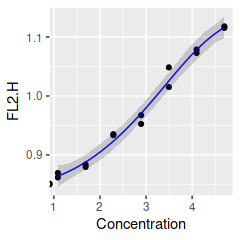### Estimate sample analyte concentration With the standard curve created we can estimate the concentrations of the samples, but also of the standards. The latter is to help us verify that the standard measurements were all fine, and that we can trust the estimation of the sample concentrations. After calculating the concentrations we can plot the known standard concentrations versus the estimated standard concentrations using the function plot_target_est_conc() and visualize where the samples fall on the standard curve with plot_target_est_conc. plex_data <- plex_data |> group_by(Analyte.ID) |> mutate(Standard data = purrr::map2(Standard data, Model fit, calculate_concentration)) |> mutate(Sample data = purrr::map2(Sample data, Model fit, calculate_concentration)) |> mutate(Std conc = purrr::map(Standard data, plot_target_est_conc)) |> mutate(Est curve = purrr::pmap(list(Sample data, Standard data, Model fit, name), plot_estimate)) I prefer to see the standard curve and the correlation of the calculated standard concentration and known standard concentration side by side: comb_plots <- function(..., .title, .ncol, .nrow = 1){ .grobs <- list(...) if(missing(.ncol)){ .ncol <- length(.grobs) } gridExtra::marrangeGrob(grobs = .grobs, ncol = .ncol, nrow = .nrow, top = .title) } plex_data <- plex_data |> mutate(Std plots = pmap(list(Std curve, Std conc, .title = name), comb_plots)) Here we look at the results for Angiopoietin-2: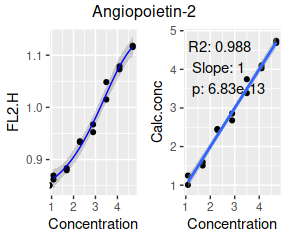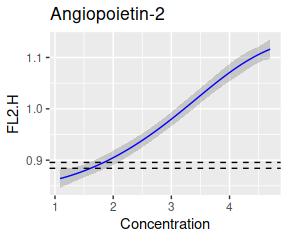With all the relevant plots in a single column they can be saved to a single file: plots_to_save <- gridExtra::marrangeGrob(plex_data$Std plots |> flatten(),
ncol = 1, nrow = 6)

ggsave("std_plots.pdf", plot = plots_to_save,
path = "./", width = 210, height = 297, units = "mm",
title = "Standard plots")

We should also not forget to save the visualize of the samples on the standard curve.

plots_to_save <- gridExtra::marrangeGrob(plex_data\$Est curve,
ncol = 1, nrow = 6)

ggsave("estimation_curve.pdf", plot = plots_to_save,
path = "./", width = 210, height = 297, units = "mm",
title = "Samples on std curve")

Lastly we fulfill the purpose of everything above - we extract the concentration of each analyte for each sample

plex_data |>
unnest(Sample data) |>
mutate(Calc.conc = 10^Calc.conc, Calc.conc error = 10^Calc.conc error) 

## Session info

## R version 4.1.2 (2021-11-01)
## Platform: x86_64-pc-linux-gnu (64-bit)
## Running under: Ubuntu 21.04
##
## Matrix products: default
## BLAS:   /usr/lib/x86_64-linux-gnu/blas/libblas.so.3.9.0
## LAPACK: /usr/lib/x86_64-linux-gnu/lapack/liblapack.so.3.9.0
##
## locale:
##   LC_CTYPE=en_US.UTF-8       LC_NUMERIC=C
##   LC_TIME=de_DE.UTF-8        LC_COLLATE=C
##   LC_MONETARY=de_DE.UTF-8    LC_MESSAGES=en_US.UTF-8
##   LC_PAPER=de_DE.UTF-8       LC_NAME=C
##  LC_MEASUREMENT=de_DE.UTF-8 LC_IDENTIFICATION=C
##
## attached base packages:
##  stats     graphics  grDevices utils     datasets  methods   base
##
## other attached packages:
##  tidyr_1.2.0     stringr_1.4.0   gridExtra_2.3   purrr_0.3.4
##
## loaded via a namespace (and not attached):
##   mclust_5.4.9     Rcpp_1.0.8       mvtnorm_1.1-3    lattice_0.20-45
##   zoo_1.8-9        gtools_3.9.2     digest_0.6.29    utf8_1.2.2
##   R6_2.5.1         evaluate_0.15    highr_0.9        pillar_1.7.0
##  rlang_1.0.1      multcomp_1.4-18  rstudioapi_0.13  car_3.0-12
##  raster_3.5-15    jquerylib_0.1.4  hexbin_1.28.2    Matrix_1.4-0
##  rmarkdown_2.12   splines_4.1.2    labeling_0.4.2   igraph_1.2.11
##  munsell_0.5.0    compiler_4.1.2   xfun_0.30        pkgconfig_2.0.3
##  mgcv_1.8-39      htmltools_0.5.2  tidyselect_1.1.2 tibble_3.1.6
##  codetools_0.2-18 fansi_1.0.2      crayon_1.5.0     withr_2.5.0
##  MASS_7.3-55      grid_4.1.2       nlme_3.1-155     jsonlite_1.8.0
##  gtable_0.3.0     lifecycle_1.0.1  magrittr_2.0.2   scales_1.1.1
##  cli_3.2.0        stringi_1.7.6    carData_3.0-5    farver_2.1.0
##  sp_1.4-6         bslib_0.3.1      ellipsis_0.3.2   drc_3.0-1
##  generics_0.1.2   vctrs_0.3.8      sandwich_3.0-1   TH.data_1.1-0
##  tools_4.1.2      glue_1.6.2       plotrix_3.8-2    abind_1.4-5
##  fastmap_1.1.0    survival_3.3-1   yaml_2.3.5       colorspace_2.0-3
##  terra_1.5-21     cluster_2.1.2    knitr_1.37       sass_0.4.0